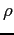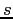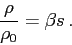Next: Specifying boundary conditions Up: Specifying initial and boundary Previous: Specifying initial and boundary   Contents

## Initial conditions

Initial conditions are set by altering the functions in the file initialization.c so that they return the desired initial distributions. There are five functions that specify the initial depth, free-surface, salinity, temperature, and velocity fields. Each function takes as its argument the x,y,z coordinates of the desired initial condition. You need to edit the function to return the desired initial value based on the given x,y,z coordinates. The five functions are

• ReturnDepth This returns the depth as a function of the given x,y coordinate. As an example, to set a linear slope that rises from 10 m depth to 1 m depth in 1000 m, the function would look like
REAL ReturnDepth(REAL x, REAL y) {
return 10 - 9*x/1000;
}

Note that the depth is specified as a positive quantity. This function is only used when the IntDepth variable is set to 0 in suntans.dat. Otherwise, when IntDepth is set to 1, the depth is interpolated from the file specified by the depth file in suntans.dat. This file must contain the depth data in three columns given by x, y, depth, where depth is specified as a negative quantity and elevation is positive.
• ReturnFreeSurface This returns the initial free surface distribution as a function of the x,y coordinate. As an example, to initialize the free surface with a 1 m cosine seiche in a 1000 m domain, the function would look like
REAL ReturnFreeSurface(REAL x, REAL y, REAL d) {
return cos(PI*x/1000);
}

Note that PI is a global variable defined in the file suntans.h as 3.141592654. The depth is also supplied to the ReturnFreeSurface function in case the free surface is a function of the depth.
• ReturnSalinity and ReturnTemperature These are similar functions in that they return the specified scalar field as a function of the x, y, and z coordinates. Note that if beta is 0 in suntans.dat, then salinity transport is not computed, likewise if gamma is 0, then temperature transport is not computed. As it is now, the code computes the temperature as a passive scalar while the density anomalyis computed solely as a function of the salinity anomaly, such thatThis equation of state is specified in the file state.c.
• ReturnHorizontalVelocity This returns the horizontal velocity defined at the faces of each cell. The initial vertical velocity is computed by continuity from this initial velocity field. Since this function returns the velocity normal to a cell face, then you must specify both velocity components u and v and then return the component normal to the face, which is defined by the vector with components n1 and n2. As an example, to return an irrotational vortex with maximum velocity of 1, centered about (x,y)=(5,5), the function would appear as
REAL ReturnHorizontalVelocity(REAL x, REAL y, REAL n1, REAL n2, REAL z) {
REAL u, v, umag=1;

u = -umag*(y-5)/5;
v = umag*(x-5)/5;

return u*n1+v*n2;
}Next: Specifying boundary conditions Up: Specifying initial and boundary Previous: Specifying initial and boundary   Contents
2014-08-06